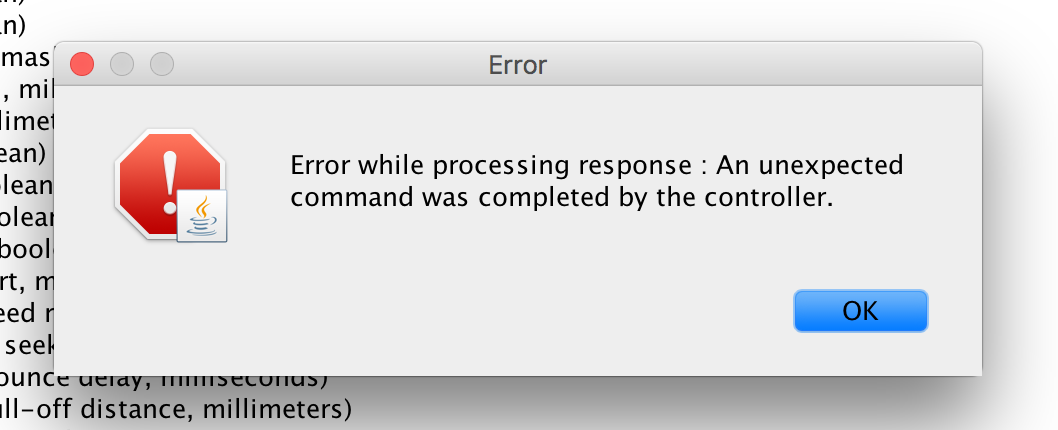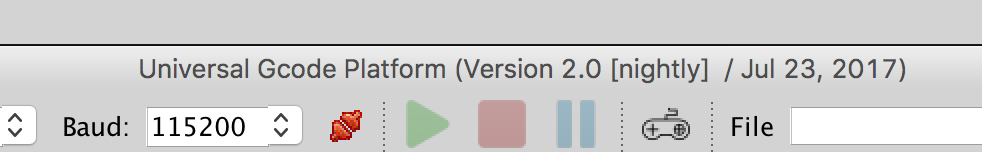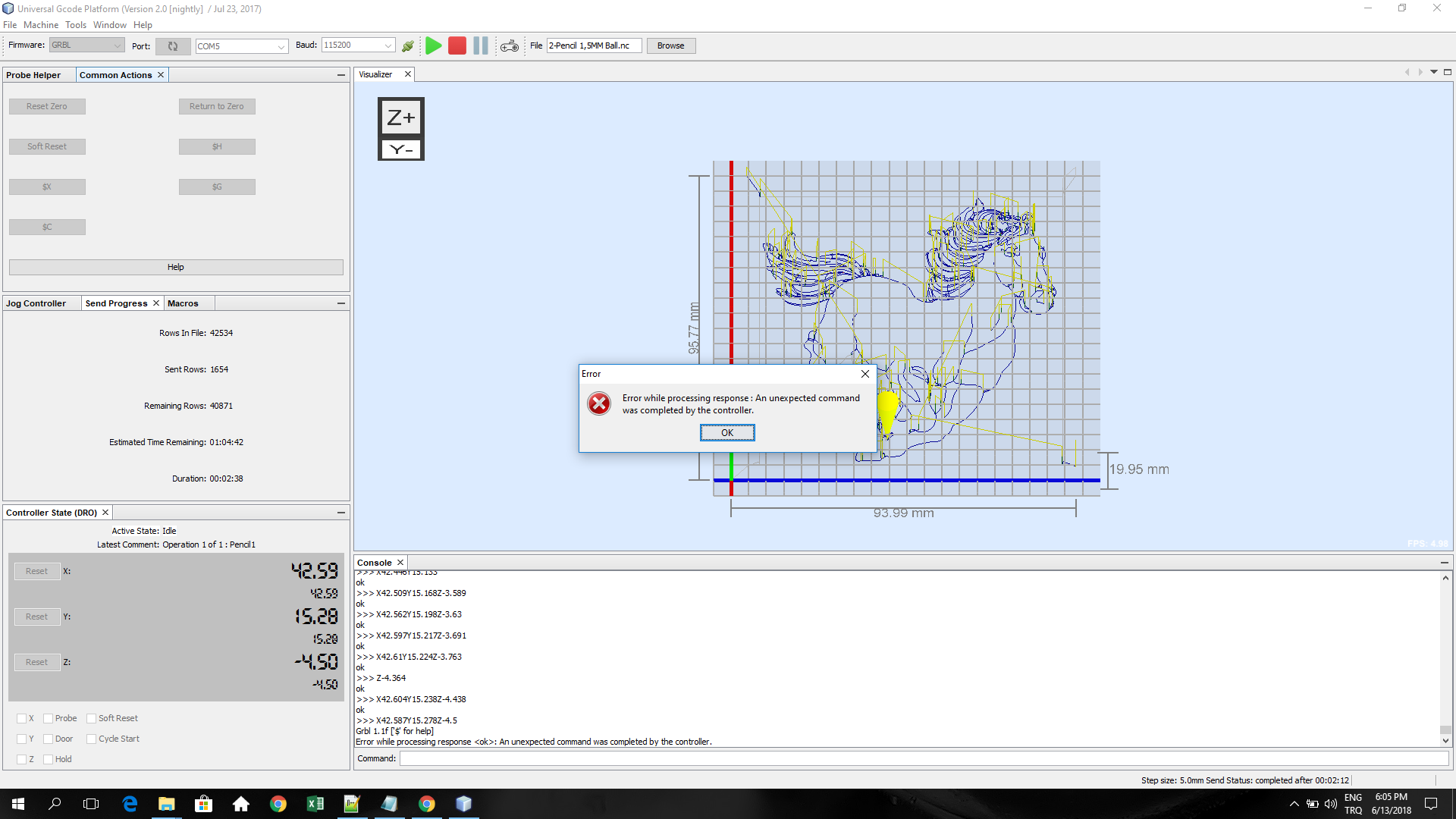开源改变世界!!

# 控制器完成了意外命令。grbl1.1f更新后 #1017

4个月前 (01-27) 89次浏览

打开了这个问题 2018 年 4 月 28 日 · 10 条评论

# 控制器完成了意外命令。grbl1.1f更新后#1017

打开了这个问题 2018 年 4 月 28 日 · 10 条评论

## 问题描述

`Error while processing response <ok>: An unexpected command was completed by the controller.`

### 实际行为1. 打开程序
2. 单击串行连接按钮
3. 出现错误信息

### 规格

#### 版本

Java：1.8.0_121；Java HotSpot(TM) 64 位服务器 VM 25.121-b13

#### 操作系统

Mac OS X 版本 10.13.4

GRBL 1.1f

#### 其他

``````**** Connected to /dev/cu.wchusbserial1410 @ 115200 baud ****
Grbl 1.1f ['\$' for help]
>>> \$\$
\$0 = 10    (Step pulse time, microseconds)
\$1 = 25    (Step idle delay, milliseconds)
\$2 = 0    (Step pulse invert, mask)
\$3 = 5    (Step direction invert, mask)
\$4 = 0    (Invert step enable pin, boolean)
\$5 = 0    (Invert limit pins, boolean)
\$6 = 0    (Invert probe pin, boolean)
\$10 = 3    (Status report options, mask)
\$11 = 0.010    (Junction deviation, millimeters)
\$12 = 0.002    (Arc tolerance, millimeters)
\$13 = 0    (Report in inches, boolean)
\$20 = 0    (Soft limits enable, boolean)
\$21 = 0    (Hard limits enable, boolean)
\$22 = 0    (Homing cycle enable, boolean)
\$23 = 0    (Homing direction invert, mask)
\$24 = 25.000    (Homing locate feed rate, mm/min)
\$25 = 500.000    (Homing search seek rate, mm/min)
\$26 = 250    (Homing switch debounce delay, milliseconds)
\$27 = 1.000    (Homing switch pull-off distance, millimeters)
\$30 = 1000    (Maximum spindle speed, RPM)
\$31 = 0    (Minimum spindle speed, RPM)
\$32 = 0    (Laser-mode enable, boolean)
\$100 = 800.000    (X-axis travel resolution, step/mm)
\$101 = 800.000    (Y-axis travel resolution, step/mm)
\$102 = 800.000    (Z-axis travel resolution, step/mm)
\$110 = 5000.000    (X-axis maximum rate, mm/min)
\$111 = 5000.000    (Y-axis maximum rate, mm/min)
\$112 = 800.000    (Z-axis maximum rate, mm/min)
\$120 = 10.000    (X-axis acceleration, mm/sec^2)
\$121 = 10.000    (Y-axis acceleration, mm/sec^2)
\$122 = 10.000    (Z-axis acceleration, mm/sec^2)
\$130 = 200.000    (X-axis maximum travel, millimeters)
\$131 = 200.000    (Y-axis maximum travel, millimeters)
\$132 = 200.000    (Z-axis maximum travel, millimeters)
ok
>>> \$G
[GC:G0 G54 G17 G21 G90 G94 M5 M9 T0 F0 S0]
ok
**** Connection closed ****
15,128|FS:0,0>
**** Connected to /dev/cu.wchusbserial1410 @ 115200 baud ****
Grbl 1.1f ['\$' for help]
>>> \$\$
Grbl 1.1f ['\$' for help]
>>> \$G
>>> \$\$
\$0 = 10    (Step pulse time, microseconds)
\$1 = 25    (Step idle delay, milliseconds)
\$2 = 0    (Step pulse invert, mask)
\$3 = 5    (Step direction invert, mask)
\$4 = 0    (Invert step enable pin, boolean)
\$5 = 0    (Invert limit pins, boolean)
\$6 = 0    (Invert probe pin, boolean)
\$10 = 3    (Status report options, mask)
\$11 = 0.010    (Junction deviation, millimeters)
\$12 = 0.002    (Arc tolerance, millimeters)
\$13 = 0    (Report in inches, boolean)
\$20 = 0    (Soft limits enable, boolean)
\$21 = 0    (Hard limits enable, boolean)
\$22 = 0    (Homing cycle enable, boolean)
\$23 = 0    (Homing direction invert, mask)
\$24 = 25.000    (Homing locate feed rate, mm/min)
\$25 = 500.000    (Homing search seek rate, mm/min)
\$26 = 250    (Homing switch debounce delay, milliseconds)
\$27 = 1.000    (Homing switch pull-off distance, millimeters)
\$30 = 1000    (Maximum spindle speed, RPM)
\$31 = 0    (Minimum spindle speed, RPM)
\$32 = 0    (Laser-mode enable, boolean)
\$100 = 800.000    (X-axis travel resolution, step/mm)
\$101 = 800.000    (Y-axis travel resolution, step/mm)
\$102 = 800.000    (Z-axis travel resolution, step/mm)
\$110 = 5000.000    (X-axis maximum rate, mm/min)
\$111 = 5000.000    (Y-axis maximum rate, mm/min)
\$112 = 800.000    (Z-axis maximum rate, mm/min)
\$120 = 10.000    (X-axis acceleration, mm/sec^2)
\$121 = 10.000    (Y-axis acceleration, mm/sec^2)
\$122 = 10.000    (Z-axis acceleration, mm/sec^2)
\$130 = 200.000    (X-axis maximum travel, millimeters)
\$131 = 200.000    (Y-axis maximum travel, millimeters)
\$132 = 200.000    (Z-axis maximum travel, millimeters)
ok
[GC:G0 G54 G17 G21 G90 G94 M5 M9 T0 F0 S0]
ok
>>> \$G
\$0 = 10    (Step pulse time, microseconds)
\$1 = 25    (Step idle delay, milliseconds)
\$2 = 0    (Step pulse invert, mask)
\$3 = 5    (Step direction invert, mask)
\$4 = 0    (Invert step enable pin, boolean)
\$5 = 0    (Invert limit pins, boolean)
\$6 = 0    (Invert probe pin, boolean)
\$10 = 3    (Status report options, mask)
\$11 = 0.010    (Junction deviation, millimeters)
\$12 = 0.002    (Arc tolerance, millimeters)
\$13 = 0    (Report in inches, boolean)
\$20 = 0    (Soft limits enable, boolean)
\$21 = 0    (Hard limits enable, boolean)
\$22 = 0    (Homing cycle enable, boolean)
\$23 = 0    (Homing direction invert, mask)
\$24 = 25.000    (Homing locate feed rate, mm/min)
\$25 = 500.000    (Homing search seek rate, mm/min)
\$26 = 250    (Homing switch debounce delay, milliseconds)
\$27 = 1.000    (Homing switch pull-off distance, millimeters)
\$30 = 1000    (Maximum spindle speed, RPM)
\$31 = 0    (Minimum spindle speed, RPM)
\$32 = 0    (Laser-mode enable, boolean)
\$100 = 800.000    (X-axis travel resolution, step/mm)
\$101 = 800.000    (Y-axis travel resolution, step/mm)
\$102 = 800.000    (Z-axis travel resolution, step/mm)
\$110 = 5000.000    (X-axis maximum rate, mm/min)
\$111 = 5000.000    (Y-axis maximum rate, mm/min)
\$112 = 800.000    (Z-axis maximum rate, mm/min)
\$120 = 10.000    (X-axis acceleration, mm/sec^2)
\$121 = 10.000    (Y-axis acceleration, mm/sec^2)
\$122 = 10.000    (Z-axis acceleration, mm/sec^2)
\$130 = 200.000    (X-axis maximum travel, millimeters)
\$131 = 200.000    (Y-axis maximum travel, millimeters)
\$132 = 200.000    (Z-axis maximum travel, millimeters)
ok
[GC:G0 G54 G17 G21 G90 G94 M5 M9 T0 F0 S0]
Error while processing response <ok>: An unexpected command was completed by the controller.
``````
评论了 2018 年 4 月 29 日

 我无法在夜间构建中重现此错误。 看来你的UGS是旧版本（20160930），能不能试试最新的nightly build，看看问题是否还存在？ （顺便说一句，感谢出色的错误报告。）

## UGS 平台 – 通用 Gcode 平台，下一代基于平台的界面

``````Product Version: Universal Gcode Platform 201609300101
Java: 1.8.0_121; Java HotSpot(TM) 64-Bit Server VM 25.121-b13
Runtime: Java(TM) SE Runtime Environment 1.8.0_121-b13
System: Mac OS X version 10.13.4 running on x86_64; UTF-8; en_US (ugsplatform)
User directory: /Users/work/Library/Application Support/ugsplatform/2.0-SNAPSHOT/dev
Cache directory: /Users/work/Library/Application Support/ugsplatform/2.0-SNAPSHOT/dev/var/cache
``````

## UGS 平台 – 构建通用 Gcode 平台，下一代基于平台的接口（需要 Java 8）

``````Product Version: NetBeans Platform 8.1 (Build 201510222201)
Java: 1.8.0_121; Java HotSpot(TM) 64-Bit Server VM 25.121-b13
Runtime: Java(TM) SE Runtime Environment 1.8.0_121-b13
System: Mac OS X version 10.13.4 running on x86_64; UTF-8; en_US (serverlaunch)
User directory: /Users/work/Library/Application Support/ugsplatform/dev
Cache directory: /Users/work/Library/Application Support/ugsplatform/dev/var/cache
``````哦，对不起！关于对话框中的版本字符串未正确更新。
 我也有同样的问题。 可能与弧度设置有关。我在以前的版本中遇到 G2/G3 命令的问题，所以我从设置中选择将 G2/G3 命令更改为 G1。 我今天安装了最新版本。我导入了 gcode 文件。虽然文件中有 42000 行，但它只能识别 1450 行。这是第 1450 行的 gcode 命令： G19 G3 X42.3957 Y15.587 Z-4.9025 J0.0168 K0.042 当我回到之前启用 G2/G3 命令的 Arc Setting 时，这次所有 42000 行都被识别，但是，程序在第 1669 行给出了以下错误。 我也附上了 gcode 文件。gcode文件.zip
 我只是通过尝试 HOME 机器遇到了同样的问题
 我自己不使用 Homing。 但是您可以检查以确保它已打开。 \$\$ check \$22 和 \$130 \$131 \$132 是你的床尺寸的限制。
 嗨， 我对 nigtly build 有同样的问题。在蜡烛上（例如）- 没有问题。程序也不会一直停在同一个地方（如果重复几次）。 错误：“控制器完成了意外命令” ``````com.willwinder.universalgcodesender.AbstractController\$UnexpectedCommand: An unexpected command was completed by the controller. at com.willwinder.universalgcodesender.AbstractController.commandComplete(AbstractController.java:793) [catch] at com.willwinder.universalgcodesender.GrblController.rawResponseHandler(GrblController.java:158) at com.willwinder.universalgcodesender.AbstractController.rawResponseListener(AbstractController.java:815) at com.willwinder.universalgcodesender.AbstractCommunicator.sendEventToListeners(AbstractCommunicator.java:172) at com.willwinder.universalgcodesender.AbstractCommunicator.lambda\$new\$0(AbstractCommunicator.java:194) at java.lang.Thread.run(Thread.java:748) `````` 控制台输出： ``````ok >>> X-75.341Y-13.148 [Error] Error while processing response : An unexpected command was completed by the controller. [Error] Error while processing response : An unexpected command was completed by the controller. [Error] Error while processing response : An unexpected command was completed by the controller. [Error] Error while processing response : An unexpected command was completed by the controller. [Error] Error while processing response : An unexpected command was completed by the controller. [Error] Error while processing response : An unexpected command was completed by the controller. **** Connected to ttyUSB0 @ 57600 baud **** Grbl 1.1g ['\$' for help] ``````
 查看日志，看起来与控制器的连接不稳定。欢迎消息显示在日志中间，表示控制器已重置。检查您的 USB 电缆并确保它是优质的屏蔽电缆。 如果仍有问题，请打开一个新的新问题，我们将从那里开始。